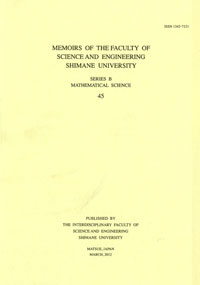# Memoirs of the Graduate School of Science and Engineering, Shimane University. Series B, Mathematics

ISSN:1342-7121number of downloads : ?
Memoirs of the Graduate School of Science and Engineering, Shimane University. Series B, Mathematics 54
2021-01-30 発行

# DISCRETE MULTI-HARMONIC GREEN FUNCTIONS

KURATA, HISAYASU
YAMASAKI, MARETSUGU
Description
The harmonic Green function ga of an infinite network defined as the unique Dirichlet potential which satisfies Δga = −δa. The biharmonic Green function ga(2) (x) is defined by the convolution of gx and ga in . It is known that Δ2ga(2) = δa if ga(2) is finite and that ga(2) is a Dirichlet potential if ga has a finite Green energy. In this paper, we define the k-harmonic Green function ga(k) (x) as the convolution of gx(k−1) and ga if it converges. We study some potential theoretic properties related to ga(k).